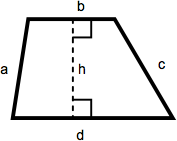## ASVAB Math Knowledge Practice Test 786524

Question 1 of 5
###### Trapezoid

A trapezoid is a quadrilateral with one set of parallel sides. The area of a trapezoid is one-half the sum of the lengths of the parallel sides multiplied by the height. In this diagram, that becomes ½(b + d)(h).The dimensions of this trapezoid are a = 6, b = 3, c = 8, d = 3, and h = 4. What is the area?

 12 42$$\frac{1}{2}$$ 10$$\frac{1}{2}$$ 16